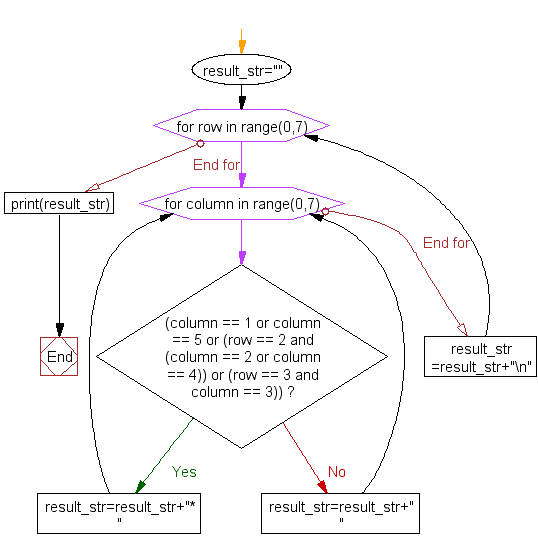﻿ Python Exercise: Print alphabet pattern M - w3resource# Python Exercise: Print alphabet pattern M

## Python Conditional: Exercise - 22 with Solution

Write a Python program to print alphabet pattern 'M'.

Pictorial Presentation:Sample Solution:

Python Code:

``````result_str="";
for row in range(0,7):
for column in range(0,7):
if (column == 1 or column == 5 or (row == 2 and (column == 2 or column == 4)) or (row == 3 and column == 3)):
result_str=result_str+"* "
else:
result_str=result_str+"  "
result_str=result_str+"\n"
print(result_str);
```
```

Sample Output:

```  *       *
*       *
* *   * *
*   *   *
*       *
*       *
*       *```

Flowchart :## Visualize Python code execution:

The following tool visualize what the computer is doing step-by-step as it executes the said program:

Python Code Editor:

Have another way to solve this solution? Contribute your code (and comments) through Disqus.

What is the difficulty level of this exercise?

Test your Python skills with w3resource's quiz

﻿

## Python: Tips of the Day

Returns a list with n elements removed from the beginning

Example:

```def tips_take(itr, n = 1):
return itr[:n]
print(tips_take([1, 2, 3], 5))
print(tips_take([1, 2, 3], 0))
```

Output:

```[1, 2, 3]
[]
```